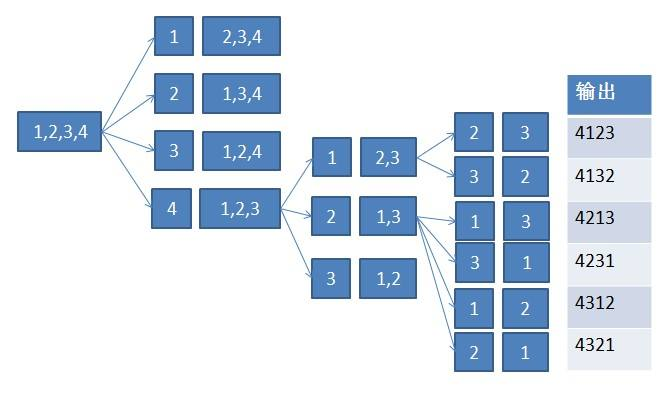# 笔试面试算法经典--全排列算法-递归&字典序实现（Java）

1 、2 、3三个元素的全排列为：

{1，2，3}，{1，3，2}，{2，1，3}，{2，3，1}，{3，1，2}，{3，2，1}。public  void Permutation(char chs[],int start )
{
if(start==chs.length-1)
{
Arrays.toString(chs);
//如果已经到了数组的最后一个元素，前面的元素已经排好，输出。
}
for(int i=start;i<=chs.length-1;i++)
{
//把第一个元素分别与后面的元素进行交换，递归的调用其子数组进行排序
Swap(chs,i,start);
Permutation(chs,start+1);
Swap(chs,i,start);
//子数组排序返回后要将第一个元素交换回来。
//如果不交换回来会出错，比如说第一次1、2交换，第一个位置为2，子数组排序返回后如果不将1、2
//交换回来第二次交换的时候就会将2、3交换，因此必须将1、2交换使1还是在第一个位置
}
}
public  void Swap(char chs[],int i,int j)
{
char temp;
temp=chs[i];
chs[i]=chs[j];
chs[j]=temp;
}


public static void Permutation(char chs[],int start)
{
if(start==end)
{
}
for(int i=start;i<=chs.length-1;i++)
{
if(i==start||chs[i]!=chs[start])
{
//在排列的时候进行判断如果后面的元素与start相同时就不进行排序。
//这样就可以避免对重复元素进行排序
Swap(chs,i,start);
Permutation(chs,start+1);
Swap(chs,i,start);
}
}
}

public  void PermutationWithDictionary(char chs[])
{
Arrays.sort(chs);
//先对数组的元素进行依次排序
while(true)
{
System.out.println(chs);
int j=chs.length-1;
int index=0;
for(j=chs.length-2;j>=0;j--)
{
if(chs[j]<chs[j+1])
{
index=j;
break;
//从右向左找到第一个非递增的元素
}
else if(j==0){
return;
}
}

for(j=chs.length-1;j>=0;j--)
{
if(chs[j]>chs[index])
break;
//从右向左找到第一个比非递增元素大的元素
}
Swap(chs,index,j);
//交换找到的两个元素
Reverse(chs,index+1);
//对非递增元素位置后面的数组进行逆序排列
}
}
public static void Reverse(char chs[],int i)
{
int k=i,j=chs.length-1;
while(k<j)
{
Swap(chs,k,j);
k++;
j--;
}
}

public static void Swap(char chs[],int i,int j)
{
char temp;
temp=chs[i];
chs[i]=chs[j];
chs[j]=temp;
}
12-3098
11-1173508-212709
02-196万+
03-2184
03-27594
03-071555
©️2020 CSDN 皮肤主题: Age of Ai 设计师:meimeiellie